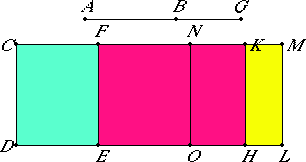# Proposition 98

The square on a first apotome of a medial straight line applied to a rational straight line produces as breadth a second apotome.

Let AB be a first apotome of a medial straight line and CD a rational straight line, and to CD let there be applied CE equal to the square on AB producing CF as breadth.

I say that CF is a second apotome.

X.74

Let BG be the annex to AB. Then AG and GB are medial straight lines commensurable in square only which contain a rational rectangle.To CD apply CH, equal to the square on AG, producing CK as breadth, and KL, equal to the square on GB, producing KM as breadth.

Therefore the whole CL equals the sum of the squares on AG. Therefore CL is also medial.

X.22

And it is applied to the rational straight line CD producing CM as breadth, therefore CM is rational and incommensurable in length with CD.

II.7

Now, since CL equals the sum of the squares on AG and GB, and, in these, the square on AB equals CE, therefore the remainder, twice the rectangle AG by GB, equals FL.

But twice the rectangle AG by GB is rational, therefore FL is rational.

X.20

And it is applied to the rational straight line FE producing FM as breadth, therefore FM is also rational and commensurable in length with CD.

Now, since the sum of the squares on AG and GB, that is, CL, is medial, while twice the rectangle AG by GB, that is, FL, is rational, therefore CL is incommensurable with FL.

But CL is to FL as CM is to FM, therefore CM is incommensurable in length with FM.

X.73

And both are rational, therefore CM and MF are rational straight lines commensurable in square only. Therefore CF is an apotome.

I say next that it is also a second apotome.

Bisect FM at N, and draw NO through N parallel to CD. Then each of the rectangles FO and NL equals the rectangle AG by GB.

Now, since the rectangle AG by GB is a mean proportional between the squares on AG and GB, the square on AG equals CH, the rectangle AG by GB equals NL, and the square on BG equals KL, therefore NL is also a mean proportional between CH and KL. Therefore CH is to NL as NL is to KL.

But CH is to NL as CK is to NM, and NL is to KL as NM is to MK, therefore CK is to NM as NM is to KM. Therefore the rectangle CK by KM equals the square on NM, that is, the fourth part of the square on FM.

X.17

Since CM and MF are two unequal straight lines, and the rectangle CK by KM, equal to the fourth part of the square on MF and deficient by a square figure, has been applied to the greater, CM, and divides it into commensurable parts, therefore the square on CM is greater than the square on MF by the square on a straight line commensurable in length with CM.

X.Def.III.2

And the annex FM is commensurable in length with the rational straight line CD set out, therefore CF is a second apotome.

Therefore, the square on a first apotome of a medial straight line applied to a rational straight line produces as breadth a second apotome.

Q.E.D.

## Guide

This proposition is used in X.111.